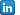April 2019

by @mw

## The fastest density estimation in R

There are many packages dealing with density estimation in R. They offer several advantages over manually coded algorithms, including bandwidth-selection procedures or involving some more complex features of density estimation, like derivative estimation or higher order kernels. Some of them are also coded in native C language, which should speed up the calculations and enhance memory management. Nevertheless, many of these extra features may be often unused in simple applications of density estimations. That leaves the question open: which algorithm is the fastest?

I try to look at the broadest possible set of R packages dealing with density estimation, including
`np`
,
`kdensity`
,
`sm`
and
`GenKern`
. There are some other packages which I skip here, as I wanted to make sure I estimate the density at a given point, and not across the domain, to make the numbers comparable. (As a side comment, package
`GenKern`
seems to have some scaling problems depending on the sample size, but given that the scaling factor is a multiple of 10, I include the package in the comparison). To finally compare the results, I write two functions to perform density estimation. The first one utilizes a standard
`for`
loop, and the second one offers a vectorized solution. In theory, the vectorized program should have quite good performance. To compare the solutions I use the package
`microbenchmark`
, which executes the codes multiple times in a random order, and compares the aggregate results.

Although there is a wide literature on density estimation, the technical implementation is quite simple. For more information and technicalities behind the method, I refer you to the set of lecture notes of Bruce Hansen, which offer a great introduction to the topic [LINK]. Given sample \$\{X_i: i=1,...,n\}\$, where \$n\$ is the sample size, and a regular kernel function \$K\$, which typically is a probability density function, one can estimate the density at point \$x\$ as

\$\$ f↖{∧}(x) = {1}/{n h} ∑↙{i=1}↖n K({x-X_i}/h). \$\$

Here, the parameter \$h\$ is the so-called bandwidth, which controls the amount of smoothing of the estimator. It is usually data-driven and converges to 0 as the sample size tends to infinity. For the experiment I take the same bandwidth parameter for all the estimators.

The strategy is simple. I would like to generate a sample of size 10,000 from a standard normal distribution, and estimate the density at point 1.25 using different algorithms. As emphasized above, I will utilize overall 6 different functions:

• K.dens - a simple function to sum over the realizations of \$X_i\$
• K.vdens - a vectorized version of K.dens
• npudens from the package
`np`
• kde from the package
`kdensity`
• sm.density from the package
`sm`
• KernSec from the package
`GenKern`

The plug-in code to reproduce the results is given below.

```#load necessary packages
require(ks)
require(np)
require(kdensity)
require(sm)
require(GenKern)
require(microbenchmark)

#non-vectorized density estimates
K.dens = function(x, H, p) {
x = as.matrix(x)
H = as.matrix(H)
n = length(x[,1])
d = length(x[1,])

dens = 0
for(i in 1:n) {
dens = dens + exp( -1/2 * t(p-x[i,]) %*% solve(H) %*% (p-x[i,]) )
}

return( dens / det(sqrt(H)) / (2*pi)^(d/2) / n )
}

#vectorized density estimates
K.vdens = function(x, H, p) {
x = as.matrix(x)
H = as.matrix(H)
d = length(x[1,])

if(d == 1) {
u = (p - x)/sqrt(H[1,1])
return( mean( dnorm( u ) / sqrt(H[1,1]) ) )
}
if(d > 1)  {
u = sweep(sweep(x,2,p),2,sqrt(diag(H)),"/")
return( mean( apply(sapply(1:d, FUN = function(i) dnorm( (p[i] - x[,i])/sqrt(H[i,i]) )/sqrt(H[i,i]) ), 1, FUN = prod) ) )
}
}

#simulation setup
vector <- rnorm(10000, 0, 1)
point  <- c(1.25)
h      <- hns(vector)
H      <- h^2

#check if the values are the same
K.dens(x = vector, H = H, p = point)
K.vdens(x = vector, H = H, p = point)
npudens(bws = h, tdat = vector, edat = point)\\$dens
kde(vector, h = h, eval.points = point)\\$estimate
sm.density(vector, h, eval.points = point)\\$estimate
KernSec(vector, xbandwidth = h, range.x=point )\\$yden
#compare the performance
ts <- microbenchmark(K.dens(x = vector, H = H, p = point),
K.vdens(x = vector, H = H, p = point),
npudens(bws = h, tdat = vector, edat = point)\\$dens,
kde(vector, h = h, eval.points = point)\\$estimate,
sm.density(vector, h, eval.points = point)\\$estimate,
KernSec(vector, xbandwidth = h, range.x=point )\\$yden)

boxplot(ts)
```

The codes generates a plot with time performance for each of the selected functions, probably similar to the graph below.As it can be observed, the kde function from
`kdensity`
package is the best but the vectorized version of the simple estimator seems to be nearly as good. Interestingly, the most advanced in my opinion
`np`
is somewhat slower, but this is a result of an extra functionality and probably many consistency checks and verifications along the lines. It may well be that the performance of these functions differs if \$X\$ vector has more dimensions, but this is a topic to be investigated in the next post.Marcin Wolski, PhDView my profileIDEAS/RePEc